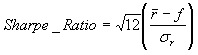Sharpe Ratio

Sharpe Ratio is a measure of risk-adjusted return.  It is desirable to have a high Sharpe Ratio, as this indicates more return for a given amount of risk.  Sharpe Ratios can be calculated for any investment, symbol, asset type, investment goal, sector, investment type, currency, or sub-portfolio.

#### Calculation Details:

When calculating the Sharpe Ratio all the monthly Time-Weighted Returns (TWR) over the specified comparison period are calculated.  For example, if the comparison period is 5 years, there are 60 monthly returns calculated.  The Sharpe Ratio is calculated using the formula:where r represents the monthly returns, and f represents a monthly risk-free return.  When calculating returns your current interpolation range preferences are used.  If sufficient pricing data is not available the Sharpe Ratio of investments will not be reported.

Fund Manager can output the data points used in this calculation to a log file.  By default the logging feature is turned off.  To turn it on, create a new registry string value at:

HKEY_CURRENT_USER\Software\Beiley Software\Fund Manager\CurrentVersion\techanal

named "benchmarklog" and set it to a value of "1".  When calculating the Sharpe Ratio for an investment the log file is named "sharpe_log.txt".  When calculating the Sharpe Ratio for a sub-portfolio the log file is named "sharpe_port_log.txt".  Each of these logs will be located in your user's AppData\Roaming\Fund Manager folder (usually a hidden folder).  These log files are deleted upon exiting Fund Manager.